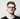# JavaScript Variables and their Scope

June 12, 2015

To understand JavaScript we must first understand two key principles, the scope of a variable and variable hoisting.

### Well What Exactly is Scope?

If we have a quick google for “define: variable scope” we are returned the following:

A variable can be either of global or local scope. A global variable is a variable declared in the main body of the source code, outside all functions, while a local variable is one declared within the body of a function or a block. However the simplest and best example I’ve seen is:

A variable’s scope is the context in which the variable exists. The scope specifies from where you can access a variable and whether you have access to the variable in that context. Variables have either a local scope or a global scope.

### Well What Exactly is Variable Hoisting?

All variables are lifted to the top of their function, where they are declared, if they are declared outside of a function then they are raised to the top of the global context, an example of variable hoisting:

``````function displayNumber () {
console.log (“First Number: “ + number); // undefined
​ var number = 1; // Set number to equal 1
console.log (“Second Number: “ + number); // 1
}``````

is translated to:

``````function displayNumber(){
var number; // number is hoisted (yet still undefined)
console.log (“First Number: “ + number); // undefined
​ number = 1; // Set number to equal 1
console.log (“Second Number: “ + number); // 1
}``````

## The Different Types of Scope

There are two main types of scope function level and block level

### Block Level

Block level scope is when the local scope of a variable is defined by the curly braces around a block of code, for example:

``````var number = 1; // Set number to 1

if (number) {
number = 2; // Set number to 2
console.log(number); // 2
}

console.log(number); // 2``````

If the above example did have block level scope, then assigning the “number” variable inside the if statement would have created a local variable “number” within that scope, instead because javascript has a function level scope it assigned the value “2” to the “number” variable

### Function Level

Function level scope is where local variables can only be defined within a function, for example:

``````var number = 1; // Set number to 1

function displayNumber(){
var number = 2; // Set number to 2
console.log(number); // 2
}

displayNumber(); // 2
console.log(number); // 1``````

As you can see in the above example the “number” variable set inside “displayNumber()” is local to that function, you can only access it in “displayNumber()”

## Best Practices

### Always Declare Local Variables

You must always declare your local variables using “var” because if you do not declare a local variable with “var” then it becomes a part of the global scope, this can cause a lot of annoying bugs, there is no reason to not do it. Lets see how this could be a problem, using a slightly modified example from above:

``````var number = 1; // Set number to 1

function displaySecretNumber(){
number = 2; // Set number to 2
console.log(number);
}

function displayNumber(){
console.log(number);
}

displayNumber(); // 1
displaySecretNumber(); // 2
displayNumber(); // 2``````

### Local Beats Global

Local variables take priority over global variables in functions, when you attempt to use a variable inside a function the local variable will take precedence:

``````var number = 1; // Set number to 1

function displayNumber(){
var number = 2; // Set number to 2
console.log(number);
}

displayNumber() // 2``````

### Stop Pollution

To be the best at JavaScript you must avoid polluting your global scope, keep global variables to a minimum, i’m not saying you’ll never need to use a global but I am saying be smart about it and use your judgement, below is an example of a polluted piece of code:

``````var number = 1 // Set number to 1

function displayNumber(){
console.log(number);
}``````

A better way to write the above piece of code is as follows:

``````function displayNumber(){
var number = 1; // Set number to 1
console.log(number);
}``````